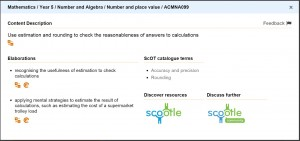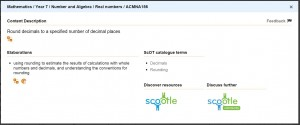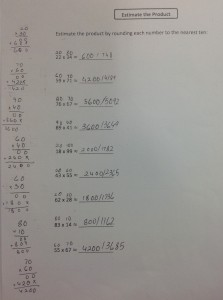Having spent 30 years in the secondary classroom, I jumped at the opportunity to visit a primary school recently. I spent some time in a couple of Grade 5 and 6 classrooms, where students were working on their ILP’s (Individual Learning Plans). One student was working on the goal of estimation, where she was asked to do the following: “Estimate the product by rounding each number to the nearest 10”.

The first problem was 22 × 34, and this particular student had written an answer of 750.

The next was 59 × 71, and this time the answer given by the student was 4180.

I was fascinated. Don’t get me wrong – it’s not like I hadn’t seen this sort of thing before. It came up often at the Year 7 and 8 level, too.

So I chatted to the student…

I needed to know if she understood the ideas of “product” and “rounding to the nearest 10”.

“Product is the same as times”, she replied. Tick. “And when you round to the nearest 10, the answer ends in a zero”. Lacking in detail, but tick.

When I asked her which of her two estimations she believed was best, she said, “The first one…because that one was only out by 2 but the other was out by 9”.

How did she know how far away from the correct answer she was?

Because…she had worked it out exactly on her page, in black and white.

She had worked out all the answers to the questions, and it was evident to me that her ability to multiply two digit numbers was excellent. But she knew that an exact answer was not required, so she worked backwards to put in a close estimate, and not all of them ended in a zero, so there was some real misconceptions happening here.

Were her estimates close to the actual answer? Sure.

Did she understand the activity? Definitely not.

So we had another look at the problems. She understood that when rounding to the nearest 10, the first problem became 20 × 30. And she knew that 2 × 3 = 6 and you needed to “add two zeroes”. She was a clever girl, but had no idea that what she had been doing was wrong, given the context of the question.

“Oh, I get it”, she said, “the next one is just 60 × 70. And that is 4200. That’s easy”.

She went on to complete the rest of the sheet and proudly ran it by me. So even though her estimations were further away from the correct answer most of the time, she knew she had mastered the goal. She had thought she was doing the right thing by supplying estimates close to the correct answer.

Ah, we do like ‘teacher pleasers’ most of the time… but not in this situation.

So, where does this concept of estimation appear in the Australian Mathematics Curriculum?

Let’s take a look at Number and Algebra in Year 5:

Uses estimation and rounding to check the reasonableness of answers to calculations (such as estimating the cost of a supermarket trolley load) – (ACMNA099).

And what happens when you click on rounding on the Content Descriptor statement?

Rounding – Glossary Term

The decimal expansion of a real number is rounded when it is approximated by a terminating decimal that has a given number of decimal digits to the right of the decimal point.

Rounding to n decimal places is achieved by removing all decimal digits beyond (to the right of) the nth digit to the right of the decimal place, and adjusting the remaining digits where necessary.

If the first digit removed (the (n+1)th digit) is less than 5, the preceding digit is not changed.

For example, 4.02749 becomes 4.027 when rounded to 3 decimal places.

If the first digit removed is greater than 5, or 5 and some succeeding digit is non-zero, the preceding digit is increased by 1. For example, 6.1234586 becomes 6.12346 when rounded to 5 decimal places.

This may look a little daunting, particularly if teachers themselves are not confident with their mathematics. What does it all mean? How would I explain it to a class?

If you wanted to round 4.02749 to 3 decimal places, you need to look at the 4th decimal place, and disregard any digits to the right of this place. Underline the digit in the 4th decimal place and cross out all digits to the right:

4.02749

The rule is this: if the digit you have underlined is between 0-4 inclusive (that is, less than 5), then cross it out and rewrite the decimal showing it correct to 3 decimal places. If the digit you underlined is between 5-9 inclusive (that is, greater than or equal to 5), then you need to add one to the digit in the 3rd decimal place:

4.02749

So, the answer, rounded to 3 decimal places is 4.027.

If 6.1234586 is rounded to 5 decimal places, then underline the digit in the 6th decimal place and cross out any digits to the right:  6.1234586. However, since the digit underlined is greater than 5, we need to add 1 to the 5 in the 5th decimal place, making the rounded answer 6.12346.

Clearly, there are conversations to be had about the “what” and “why” of all of that, but for the purpose of this exercise, I will leave it there.

Clicking the link (ACMNA099) we see the elaboration for this Content Statement:Try it now!

What happens if I explore the Scootle resources on this topic?  Here are some sites you might like to have  look at:

Rounding to the Nearest 10 (ABC ‘Splash’, 3 minute clip)

Rounding to the Nearest 100 or 1000 (Khan Academy, Interactive site)

Rounding to the Nearest 10th (Khan Academy, Interactive site)

Using Rounding to Estimate with Worded Problems (Khan Academy, Interactive site)

Estimating the Product of Two Numbers (IXL Maths, Interactive site)

It’s important to note that the ideas of estimation and rounding do not change a great deal as you move into Years 6 and 7.

Let’s have a look at ‘Number and Algebra’ in Year 6:

Adds and subtracts decimals, with and without digital technologies, and uses estimation and rounding to check the reasonableness of answers (ACMNA128)  (TIMESNA18)

Clicking on the ‘ACMNA128’, we get the elaboration for this Content Descriptor:And then again exploring the Scootle resources we have the following made available:

Rounding Decimals to the Nearest Whole Number, 10th or 100th (ABC Splash, 3 minute clip)

Moving on to ‘Number and Algebra’ in Year 7:

Rounds decimals to a specified number of decimal places (ACMNA156) (TIMESNA18)

Again, clicking on the link to the elaboration and then following the Scootle resources:Understanding Place Value for Rounding (AMSI, Interactive site)

Rounding Decimals, Teacher and Student Resources (AMSI, Interactive site)

So, there was a bit of an exploration on Estimation.

Post Script: I wandered over to another student, who had clearly mastered this whole rounding thing (although still felt the need to put down the right answer).

Wait a minute…what is that stuff on the left?…but dealing with that was a whole new ball game…